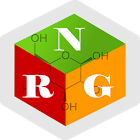Predictive Energy Calculator

All Android applications categories

All Android games categories# Predictive Energy Calculator

by: 6 9.4

9.4 Users
rating

## Screenshots

Description

The NRG Calculator is a predictive energy calculator for computing the energy needs of patients in order to support the decisions of dietitians in clinical settings.

It supports energy calculations based on a multitude of equations published in literature.

Its aim is to cover the equations that are commonly used in Canada.

Features:
• 10 equations for energy calculation
• dynamic user interface
• non-linear age input with increased precision (e.g. weeks, months) at younger ages
• body mass index (BMI), ideal body weight (IBW), and adjusted body weight (ABW) calculations